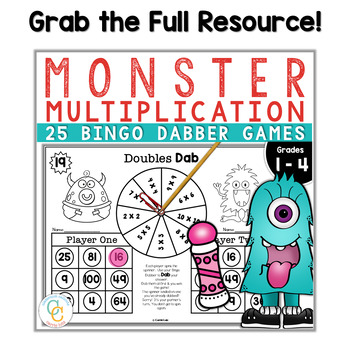# Free Bingo Dabber Multiplication Games - Distance Learning2nd - 4th, Homeschool
Subjects
Standards
Resource Type
Formats Included
• PDF
Pages
3 pages

### Description

Monster Multiplication Bingo Dabber Games will motivate your students to practice their facts. My students love using bingo dabbers. They will play math games during indoor recess if there are bingo dabbers involved! :) Fact Fluency Practice has never been more fun!

This is an easy fact fluency center. Just print, add bingo dabbers, a sharpened pencil and a paperclip and you're ready to go!

I hope your students enjoy this the way mine do!

You get:

• Times 2 Page
• Times 5 Page
• Times 10 Page

It's a great place to start since these seem to be easier to remember!

Get the entire product here.

Monster Multiplication ~ 25 Print and Play Bingo Dabber Multiplication Games

More Multiplication Practice:

Total Pages
3 pages
N/A
Teaching Duration
N/A
Report this Resource to TpT
Reported resources will be reviewed by our team. Report this resource to let us know if this resource violates TpT’s content guidelines.

### Standards

to see state-specific standards (only available in the US).
Apply properties of operations as strategies to multiply and divide. Examples: If 6 × 4 = 24 is known, then 4 × 6 = 24 is also known. (Commutative property of multiplication.) 3 × 5 × 2 can be found by 3 × 5 = 15, then 15 × 2 = 30, or by 5 × 2 = 10, then 3 × 10 = 30. (Associative property of multiplication.) Knowing that 8 × 5 = 40 and 8 × 2 = 16, one can find 8 × 7 as 8 × (5 + 2) = (8 × 5) + (8 × 2) = 40 + 16 = 56. (Distributive property.)
Determine the unknown whole number in a multiplication or division equation relating three whole numbers. For example, determine the unknown number that makes the equation true in each of the equations 8 × ? = 48, 5 = __ ÷ 3, 6 × 6 = ?.
Use multiplication and division within 100 to solve word problems in situations involving equal groups, arrays, and measurement quantities, e.g., by using drawings and equations with a symbol for the unknown number to represent the problem.
Interpret products of whole numbers, e.g., interpret 5 × 7 as the total number of objects in 5 groups of 7 objects each. For example, describe a context in which a total number of objects can be expressed as 5 × 7.